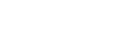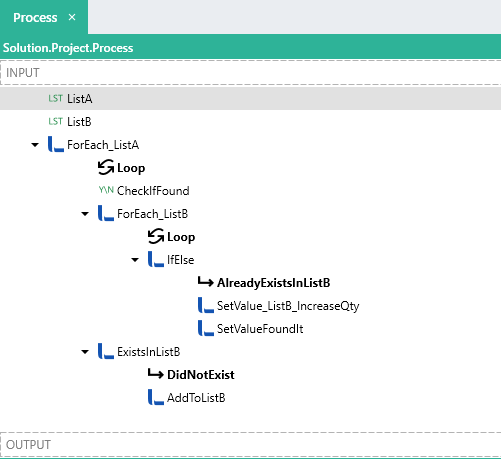# Referencing List items

## Referencing List items

When working with a ListTYP , you are able to reference specific items in lists by using the below expression:

``````= mylist[#index#].item_property
``````

This `#index#` refers the position of the item in the list and must be a IntegerTYP .

Note: All list iterations start from  that is to say the 1st item in a list would be referenced with `mylist` and the 2nd item with `mylist` etc.

However, you are only able to use the above expression when extracting certain values from a list, lets say, retrieving the first or last item in a list.

## Update specific list items

In order to set or update values of a list, you must use a ForEachFNC like below:

Let’s say you have a list like below:

``````[
{
"Name":"a",
"Quantity":3
},
{
"Name":"b",
"Quantity":2
},
{
"Name":"c",
"Quantity":1
},
{
"Name":"d",
"Quantity":2
}
]
``````

And now you want to update the `Quantity` value of the item with the `Name` of “c”.

This would be done by looping through the list using a ForEachFNC . On each iteration, a condition would then be evaluated (using an IfElseFNC ) that checks if the current loop item is equal to a value such as “c” , like the below:

``````= ForEach.Loop.Name== "c"
``````

If the condition is met, a SetValueFNC is used to set the current `ForEach.Loop.Quantity` value like below:

Target : `= ForEach.Loop.Quantity`

Source : `= ForEach.Loop.Quantity+1`

In this case, a `Quantity` field of a list item is increased.

This will mean that the item in the list of the current iteration will be updated with a new value.

Sample:

In this Solution.lsoz (5.5 KB) , unique values are counted and updated in a list.A list (`ListA`) contains multiple non-distinct characters like the below:

``````["a","b","a","a","b","c","d","d"]
``````

A list (`ListB`) contains the structure like below:

``````[ {"Name": "x" , "Quantity" : 0  } ]
``````

The `ListA` is looped through (`ForEach_ListA`).

For each item in `ListA`, a BooleanTYP (`CheckIfFound`) is reset to `False`, this will contain the result of whether or not then item already exists in `ListB`.

For each item in `ListA`, a loop is then performed on `ListB` (`ForEach_ListB`) which filters for an item with the `Name` matching the current item of `ListA` i.e. “a”, “c” ,“d” etc ,with the below expression:

``````= ForEach_ListA.Loop == ForEach_ListB.Loop.Name
``````

If the condition is met, the `Quantity` value of the current `ListB` item is then increased with a SetValueFNC .

Target : `= ForEach_ListB.Loop.Quantity`
Source : `= ForEach_ListB.Loop.Quantity + 1`

The value of `CheckIfFound` is then set to `True`.

If the value of `CheckIfFound` is equal to `False`, then a new list item is added to `ListB`.

The result will be `ListB` will be the below:

``````[
{
"Name":"a",
"Quantity":3
},
{
"Name":"b",
"Quantity":2
},
{
"Name":"c",
"Quantity":1
},
{
"Name":"d",
"Quantity":2
}
]

``````